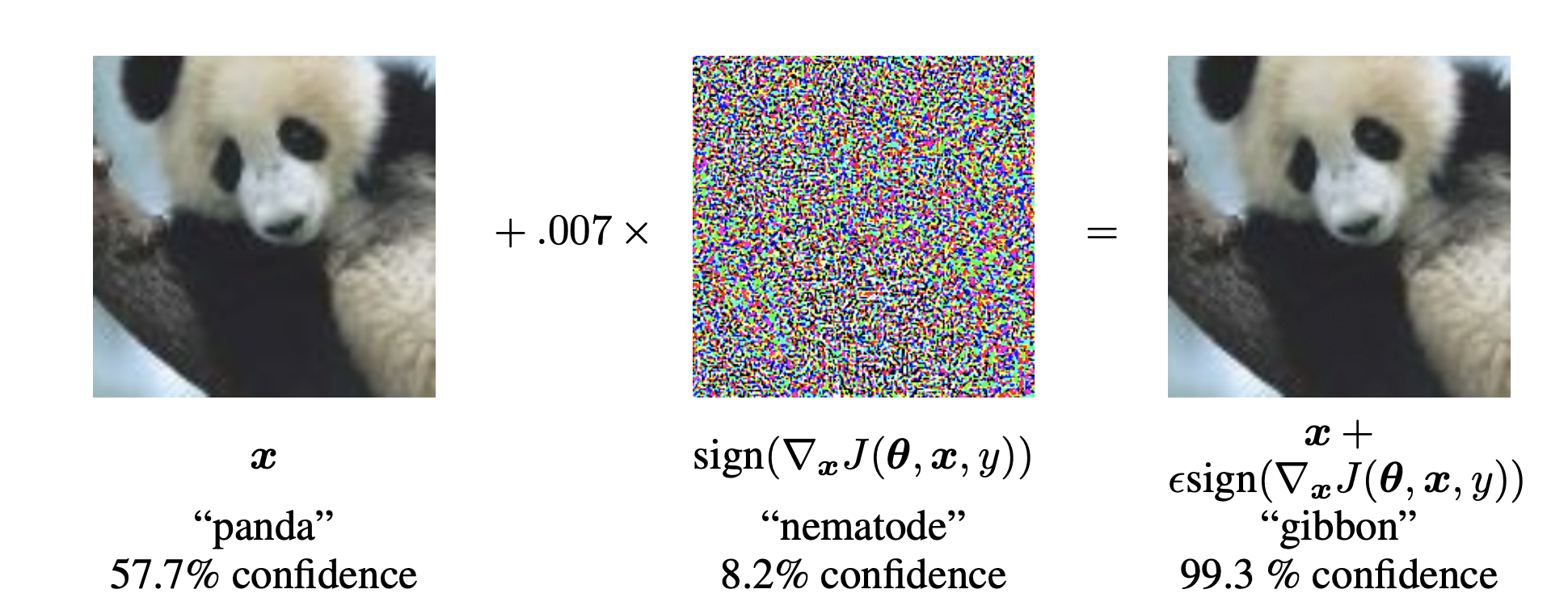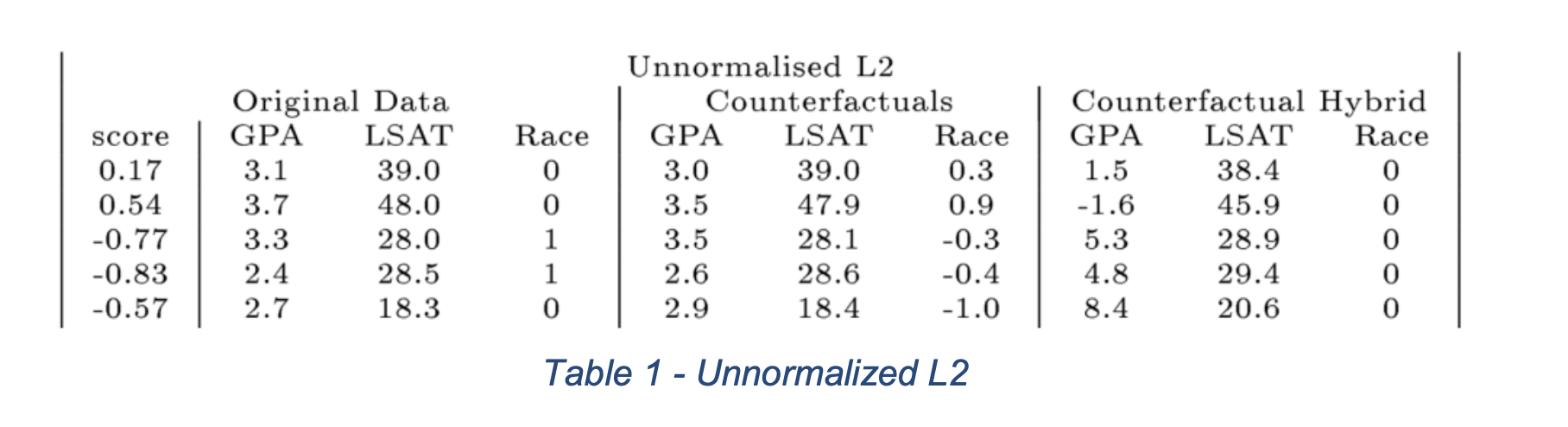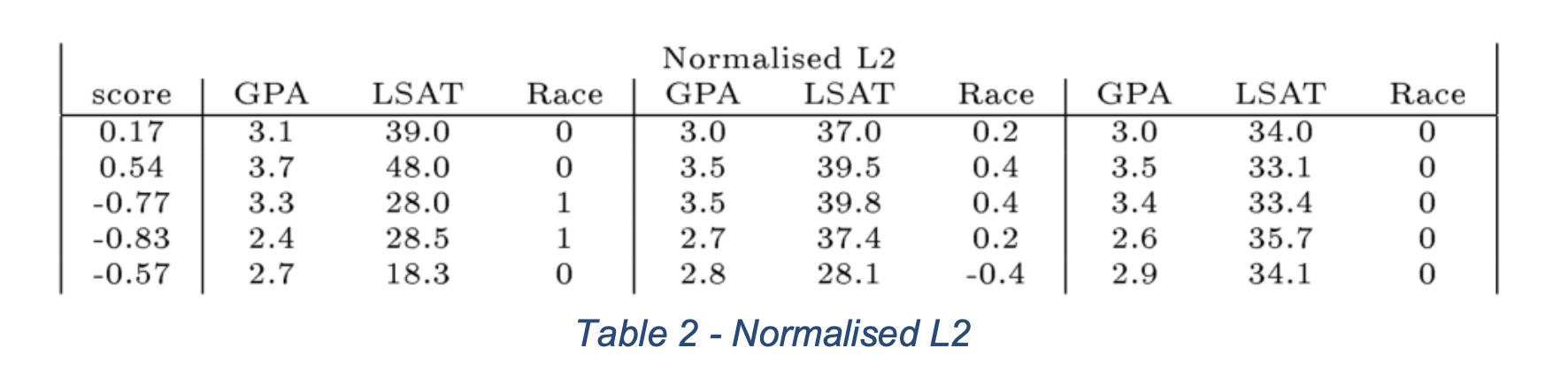# Counterfactual Explanations

Published:

## Counterfactual Explanations

“You were denied a loan because your annual income was £30,000. If your income had been £45,000, you would have been offered a loan.”

They define Counterfactual Explanations as statements taking the form:

“Score p was returned because variables V had values (v1,v2,…) associated with them. If V instead had values (v1’,v2’,…), and all other variables had remained constant, score p’ would have been returned.”

## Adversarial Examples versus Counterfactual Examples

• $x_{adv}=x+\epsilon \times\text{sign(} \nabla f_{x}(x,y)\text{ )}$
• Invisible to human eyes (see the Figure below.).(Adversarial Example generated using FGSM method. Img Src. )

### Counterfactual Example

• $x_{c}=x+v$, where $v$ only modifies few variables to $x$.

## Problem Formulation

Given function $f: X \mapsto Y$ and $n$ number of sample points, $(x_1,y_1),\dots,(x_n,y_n)$ sampled i.i.d. from distribution $P(X,Y)$, for each $(x_i,y_i)$, we want to find the counterfactual example $(x^c_i,y^c_i)$ such that,

1. $d(x^c_i,x_i) \leq \epsilon$, for some $d(\cdot,\cdot)$
2. $f(x^c_i)=y^c_i$, where $y_i \neq y^c_i$.

This can be done by solving the following optimization probblem:

$\arg\min_{x'_i} \mathcal{L}(f(x'_i),y^c_i) +d(x_i,x'_i)$

### Choice of Distance Measure

• Squared Euclidean Distance :

\begin{align} d(x_i,x^c_i) = \sum^d_{k=1} (x_{i,k} - x^c_{i,k})^2 \end{align}

• Scaled Squared Euclidean Distance :

\begin{align} d(x_i,x^c_i) = \sum^d_{k=1} \frac{(x_{i,k} - x^c_{i,k})^2}{std_k(x)} \end{align}

• Scaled L1 Norm :

\begin{align} d(x_i,x^c_i) = \sum^d_{k=1} \frac{|x_{i,k} - x^c_{i,k}|}{MAP_k} \quad \text{where,} \end{align} \begin{align} {MAP_k} = median_j (|x_{j,k} -median_l(x_{l,k})|) \end{align}

## Empirical Results

### LSAT Dataset

• prediction output : student’s first-year average grade.
• features : race, grade-point average (GPA) prior to law school, and law school entrance exam scores (LSAT).

### Counterfactual Question

“What would have to be changed to give a predicted score of 0?”

### Squared Euclidean Distance Results(Counterfactual Examples generated using Squared Euclidean Distance. Img Src. )

### Scaled Squared Euclidean Distance Results(Counterfactual Examples generated using Scaled Squared Euclidean Distance. Img Src. )

### Scaled L1 Norm Results(Counterfactual Examples generated using Squared L1 Norm. Img Src. )

• Person 1: If your LSAT was 34.0, you would have an average predicted score (0).
• Person 2: If your LSAT was 32.4, you would have an average predicted score (0).
• Person 3: If your LSAT was 33.5, and you were ‘white’, you would have an average predicted score (0).
• Person 4: If your LSAT was 35.8, and you were ‘white’, you would have an average predicted score (0).
• Person 5: If your LSAT was 34.9, you would have an average predicted score (0).

## Feasibility of Counterfactual Examples (Src.)

### Structural Causal Model (Pearl)

A causal model is a triple $M= <U,V,F>$ such that $U$ is a set of exogenous variables, $V$ is a set of endogenous variables that are determined by variables inside the model, and $F$ is a set of functions that determine the value of each $v_i \in V$ (up to some independent noise) based on values of $U_i \in U$ and $Pa_i \in V$ \ $v_i$.

### Global Feasibility

Let $<x_i,y_i>$ be the input features and the predicted outcome from $h$, and let $y'$ be the desired output class. Let $M=<U,V,F>$ be a causal model over $\mathcal{X}$ such that each feature is in $U \cup V$. Then, a counterfactual $<x^{cf},y^{cf}>$ is global feasible if i) it is valid $(y^{cf}=y')$, ii) the change from $x_i$ to $x^{cf}$ satisfies all constraints entailed by the causal model, iii) and all exogenous variabbles $x^{exog}=U$ lie within the input domain.

### Generative Model

\begin{align} \bullet \Pr(x^{cf}|y’,x) \quad \text{such that } x^{cf} \text{ belings to class } y’ \end{align}

\begin{align} \bullet \text{ encoder} \quad q(z|x,y’) \end{align}

\begin{align} \bullet \text{ decoder} \quad p(x^{cf}|z,y’,x) \end{align}

### Evidence lower bound (ELBO)

\begin{align} \ln{\Pr(x^{cf}|y’,x)} \geq \mathbb{E}_{Q(z|x,y’)} \ln{\Pr(x^{cf}|z,y’,x)} - \mathbb{KL}(Q(z|x,y’||\Pr(z|y’,x))) \end{align}

### Approximation

$\min - \mathbb{E}_{Q(z|x,y')} \ln{\Pr(x^{cf}|z,y',x)} + \mathbb{KL}(Q(z|x,y'||\Pr(z|y',x))) \\ \approx \min \mathbb{E}_{Q(z|x,y')} [\text{Dist(}x,x^{cf}\text{)} + \lambda \text{HingeLoss(}h(x^{cf}),y',\beta)\text{)} ] + \mathbb{KL}(Q(z|x,y'||\Pr(z|y',x)))$

### Causal Distance

Given causal graph $G$ over $U\cup V$ and mechanisms $F$, for each $v \in V$, we have $v=f(v_{p1},\dots,v_{pk}) + \epsilon$, where $\{v_{p1},\dots,v_{p_k}\}$ are the parents of $v$. We can define the $Distance$ for the nodes $v \in V$ as follows:

$\text{DistCausal}_v (x_v,x_v^{cf}) = \text{Distance}_v (x_v,f(x_{v_{p1}}^{cf},\dots,x_{v_{pk}}^{cf} ) )$

For the nodes $u \in U$, we can use L1 or L2 distance measure. We can now define the distance measure as follows:

$\text{Distance} (x,x^{cf}) = \sum_{u \in U} \text{Distance}_v (x_u,x_u^{cf}) + \sum_{v \in V} \text{DistCausal}_v (x_v,x_v^{cf}).$